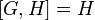# A4 in S4

This article is about a particular subgroup in a group, up to equivalence of subgroups (i.e., an isomorphism of groups that induces the corresponding isomorphism of subgroups). The subgroup is (up to isomorphism) alternating group:A4 and the group is (up to isomorphism) symmetric group:S4 (see subgroup structure of symmetric group:S4).
The subgroup is a normal subgroup and the quotient group is isomorphic to cyclic group:Z2.
VIEW: Group-subgroup pairs with the same subgroup part | Group-subgroup pairs with the same group part| Group-subgroup pairs with the same quotient part | All pages on particular subgroups in groups

This article describes the subgroup$H$ in the group$G$, where$G$ is the symmetric group of degree four, acting on the set$\{ 1,2,3,4 \}$ (for concreteness) and$H$ is the alternating group of degree four, i.e., the subset of$G$ comprising the even permutations.$H$ is a subgroup of index two, and its unique other coset (which is both a left coset and right coset) is the set of odd permutations.

Explicitly:$\! H = \{ (), (1,2)(3,4), (1,3)(2,4), (1,4)(2,3), (1,2,3), (1,3,2), (1,3,4), (1,4,3), (1,2,4), (1,4,2), (2,3,4), (2,4,3) \}$

See also element structure of symmetric group:S4 (to understand more about the elements, and which of them are even and which are odd permutations) and subgroup structure of symmetric group:S4.

## Cosets$H$ is a subgroup of index two, hence a normal subgroup. It has exactly two cosets: the subgroup itself and the rest of the group. Each of these is both a left coset and a right coset. The subgroup is the set of even permutations, and the other coset is the set of odd permutations. Explicitly:$\! H = \{ (), (1,2)(3,4), (1,3)(2,4), (1,4)(2,3), (1,2,3), (1,3,2), (1,3,4), (1,4,3), (1,2,4), (1,4,2), (2,3,4), (2,4,3) \}$$\! G \setminus H = \{ (1,2), (1,3), (1,4), (2,3), (2,4), (3,4), (1,2,3,4), (1,4,3,2), (1,2,4,3), (1,3,4,2), (1,4,2,3), (1,3,2,4) \}$

For more on the element structure and interaction with conjugacy class structure, see element structure of symmetric group:S4#Interpretation as symmetric group.

## Complements

COMPLEMENTS TO NORMAL SUBGROUP: TERMS/FACTS TO CHECK AGAINST:
TERMS: permutable complements | permutably complemented subgroup | lattice-complemented subgroup | complemented normal subgroup (normal subgroup that has permutable complement, equivalently, that has lattice complement) | retract (subgroup having a normal complement)
FACTS: complement to normal subgroup is isomorphic to quotient | complements to abelian normal subgroup are automorphic | complements to normal subgroup need not be automorphic | Schur-Zassenhaus theorem (two parts: normal Hall implies permutably complemented and Hall retract implies order-conjugate)

There are six different candidates for a permutable complement to$H$ in$G$. Since$H$ is a normal subgroup of$G$, these are also precisely the lattice complements of$H$ in$G$. Each of these is isomorphic to the quotient group cyclic group:Z2 and in fact, in this case, they are all conjugate subgroups in$G$:$\! \{ (), (1,2) \}, \{ (), (1,3) \}, \{ (), (1,4) \}, \{ (), (2,3) \}, \{ (), (2,4) \}, \{ (), (3,4) \}$

## Subgroup-defining functions

The subgroup is a characteristic subgroup and arises as a result of many common subgroup-defining functions on the whole group. Some of these are given below:

Subgroup-defining function Meaning in general Why it takes this value
derived subgroup (also called commutator subgroup) subgroup generated by commutators All the even permutations are commutators. In general, the alternating group is the derived subgroup of the corresponding symmetric group.
subgroup generated by squares subgroup generated by squares The squares are precisely the elements in the subgroup. The identity element is its own square, each 3-cycle is the square of its inverse, and each double transposition is the square of a 4-cycle.
hypocenter stable member of lower central series$H$ is the derived subgroup of$G$, and$[G,H] = H$.
Jacobson radical intersection of all maximal normal subgroups It is the unique maximal normal subgroup. The whole group is a one-headed group and this subgroup is the head.
3-Sylow closure normal closure of any 3-Sylow subgroup, or join of all the 3-Sylow subgroups

## Description in alternative interpretations of the whole group

Interpretation of$G$ Corresponding interpretation of$H$
As the symmetric group of degree four. alternating group of degree four.
As the projective general linear group of degree two over field:F3 projecitve special linear group of degree two over field:F3
As the full tetrahedral group, i.e., the full group of symmetries of the regular tetrahedron Orientation-preserving symmetries of the regular tetrahedron.
As the group of orientation-preserving symmetries of the cube or octahedron.  ?
The triangle group with parameters$(3,3,2)$ The corresponding von Dyck group with parameters$(3,3,2)$
The von Dyck group with parameters$(4,3,2)$  ?

## Subgroup properties

### Invariance under automorphisms and endomorphisms: properties

Property Meaning Satisfied? Explanation Comment
normal subgroup equals all conjugate subgroups Yes index two implies normal
characteristic subgroup invariant under all automorphisms Yes
fully invariant subgroup invariant under all endomorphisms Yes
coprime automorphism-invariant subgroup invariant under automorphisms of order coprime to the group Yes
cofactorial automorphism-invariant subgroup invariant under automorphisms whose order has no prime factors other than those in the order of the group Yes
subgroup-cofactorial automorphism-invariant subgroup invariant under automorphisms whose order has no prime factors other than those in the order of the subgroup Yes

### Advanced properties related to invariance/resemblance

Property Meaning Satisfied? Explanation Comment
verbal subgroup Yes
isomorph-free subgroup no other isomorphic subgroup Yes
isomorph-containing subgroup contains any isomorphic subgroup Yes
order-unique subgroup, index-unique subgroup no other subgroup of that order, respectively index. Order-unique and index-unique are equivalent properties in finite groups. Yes
normal subgroup having no nontrivial homomorphism to its quotient group Yes
homomorph-containing subgroup contains any homomorphic image of it in the whole group Yes
variety-containing subgroup No